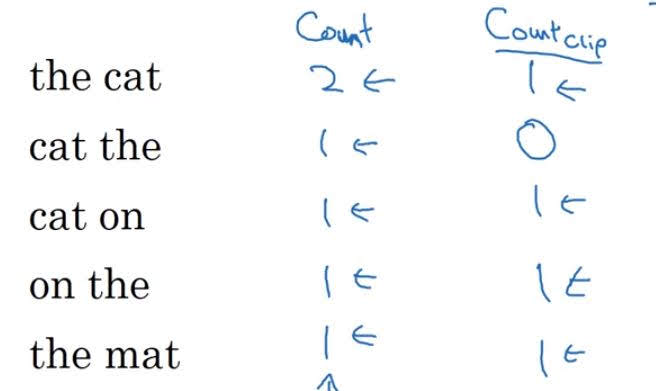# Bleu Score

Categories:

Updated:

Bleu score is a real number evaluation metric for machine translation or image captioning, where correct label can be more than one.

So long as the machine generated translation is pretty close to any of the references provided by humans, then it will get a high Bleu score.

Bleu score can be applied with respect to n-grams. This is how bleu score with $n$ gram is computed;

where $count(ngram)$ is indicates how many times a ngram appears in MT(machine translation) output, and $count_{clip}(ngram)$ indicates maximum number of times a ngram appears in one of the references.

Let’s look at an example.

• input: ‘Le chat est sur le tapis.’
• Reference 1: ‘The cat is on the mat.’
• Reference 2: ‘There is a cat on the mat.’
• MT output: ‘the cat the cat on the mat.’

Bleu score on unigrams is as follows;

Counts for bigrams are as follows;# Combined Bleu Score

For final bleu score, we combine multiple bleu scores on multiple ngrams. In the below formula, we’ve taken unigram to 4gram into account

$BP$ is a term to penalize short outputs, since short translations will be more likely to have higher bleu scores.

$BP=1$ if MT_output_length > reference_output_length

$BP=$ exp(1 - MT_output_length / reference_output_length) otherwise

Categories: